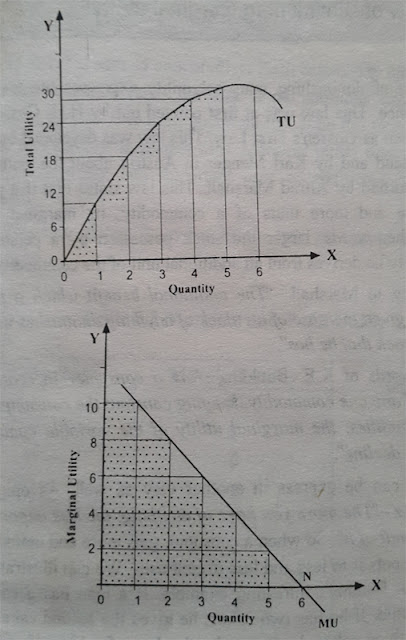## Theory of Consumer Behavior

Those who buy goods and services for their own consumption are called consumers in economics. The study of the behavior of the individual consumer is called the theory of consumer behavior. There are two approaches to the theory of consumer behavior i.e. consumer behavior – cardinal utility and ordinal utility. The concept of cardinal utility was popularized by the classical economists of late 18th and 19th centuries. The 20th century version of this theory is known as neo-classical utility theory. The concept of ordinal utility was popularized by the economists like J. R. Hicks and R.G.D. Allen. The cardinal theory assumes that utility is measurable like 1, 2, and 3. But the supporters of ordinal theory assume that ‘Quantities of utility are inherently immeasurable, theoretically and conceptually as well as practically.’ According to them utilities or satisfaction can be only ranked as 1st, 2nd, 3rd and so on. Both these theory study about consumer behavior.

An important common feature of these two approaches is that they assume that the consumer behaves rationally. It means, “The consumer calculates deliberately, chooses consistently, and maximizes utility.” The consumer attempts to maximize satisfaction from given amount of money. When they succeed we say that they have achieved optimum position.

## Total Utility and Marginal Utility

The power of a commodity to satisfy human wants is called utility. The satisfaction that consumer derives from the goods they buy is called their utility. A consumer may like orange more than mango. Because, orange has more utility. This property is common to all commodities wanted by a person. Hence, utility resides in the minds of the computer. The consumer knows the utility by introspection.

The concept of utility is ethically neutral. If someone wants it, a good or service has utility for that person. The consumption of drug may be harmful or immoral. But it has a utility, since it satisfies the want of a consumer.

The word ‘marginal’ has been used in economic theory for many decades. It means the rate of change of total. Hence, marginal utility means utility derived from the marginal or last unit.

According to Watson and Getz- “The marginal utility (MU) of any quantity n is the total utility (TU) of that quantity minus the total utility of one less. Thus, MU of n = TU of n – TU of (n – 1)”

On the other hand, total utility is the utility derived from total units consumed. As quantity consumed increases, total utility increase. Because two units of a commodity yield more utility, three unit yield still more and so on. Marginal utility is defined as the net addition made to total utility by the consumption of additional unit. The concept of total utility and marginal utility can be illustrated by the help of a table below.

Total Utility and Marginal Utility
 Units of Orange Marginal Utility Total Utility 1 2 3 4 5 6 10 8 6 4 2 0 10 18 24 28 30 30

The table shows that the marginal utility derived from 1st unit of orange is 10, from 2nd is 8, from 3rd is 6 and so on. Likewise, the total utility from 1st unit is 10, from second is 18, from 3rd is 24 and so on.

As the quantity increases, TU increases. But TU increases at a diminishing rate. The successive increases become smaller and smaller.

The relation between total utility and marginal utility has been shown in the figure below:Total Utility and Marginal Utility
In the upper part of the figure, TU is the total utility curve. The total utility curve is upward sloping but the amount of the rise is less and less as more and more are consumed. Thus the marginal utility curve is declining: each successive consumption adds to total utility but each adds less than their predecessor. Hence, the TU curve shows how total utility continues to increase as more and more units are added. The lower part of the figure shows only the increase in total utility. So, MU is the marginal utility curve. It shows the marginal utilities of different units of the commodity. When the total utility is maximum (30) at point M, marginal utility is zero at point N.